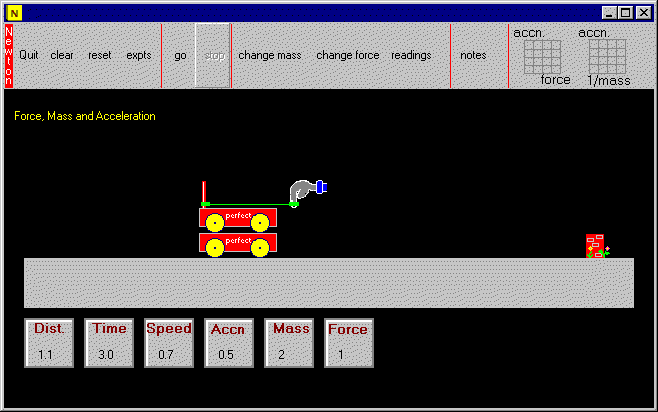html> Force Mass and Acceleration

Force, Mass and Acceleration

This program lets you apply forces to a perfectly frictionless trolley on the famous perfectly smooth surface. So we are not really on this planet. It's about Newton's second law of motion...the force is applied by pieces of stretched elastic and can be increased in steps up to 4 N.   The distance, time, speed and acceleration of the trolley are calculated for each run.  The mass is altered by piling equal trolleys on top of one another. A summary table and graphs let you see the way force, mass and acceleration are connected.  It is a simple experiment to do on a computer but difficult to do in the lab. The principles quickly become blindingly obvious but students will have to control variables properly and do a little bit of thinking on the way.